If you have a related question, please click the "Ask a related question" button in the top right corner. The newly created question will be automatically linked to this question.

# [FAQ] How do I design a-stable timer, oscillator, circuits using LMC555, TLC555, LM555, NA555, NE555, SA555, or SE555?

Other Parts Discussed in Thread: LMC555, NE555, LM555, NA555, TLC551, SE555, TLC552, TLC555, SA555, CD4060B, CD4024B, CD4040B, CD4020B

What is the difference between the part numbers?

How is the output frequency and duty cycle set?

Why is the actual frequency different from the formula, 1.44 / [(RA + 2 * RB) * C] ?

Why is the first output pulse after power up different from the subsequent pulses?

What is the maximum frequency?

What is the minimum frequency?

How accurate are the timers?

• This FAQ covers a-stable circuits. Figure 1 below shows an a-stable circuit using the LM555, NA555, NE555, SA555 and SE555 timers that are called LM, NA, NE, SA and SE respectively hereafter. As a group they are called bipolar timers due to their design. This FAQ also covers LMC555 and TLC555 timers that will be called LMC and TLC respectively hereafter. As a group they are called CMOS timers due to their design. This FAQ is applicable to the xx556, TLC551, and TLC552 timers as well. Supply voltage pins for the timers use different symbols, VS, VCC, VDD, that have the same function.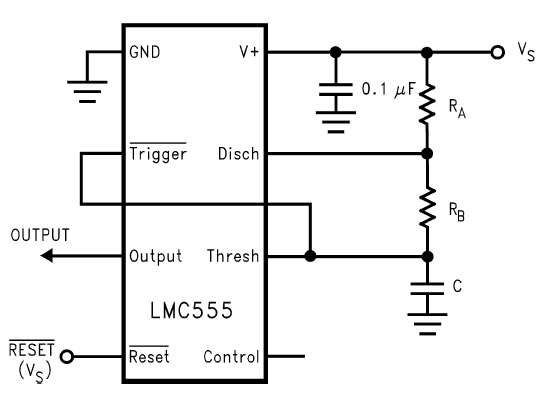Figure 1. Basic a-stable circuit

A-stable mode, as the name implies, has no stable state. The output alternates between low and high at a rate set by two resistors and a capacitor. The a-stable setup ties ‘threshold pin’, ‘trigger pin’, and timing capacitor together so that the timer retriggers itself as the capacitor voltage ramps between 1/3 VS and 2/3 VS.

Resistor (RB) is connected from the timing capacitor to the ‘discharge pin’. This sets the discharge time, where the output is low. Low time is RB * C * ln(2). Resistor (RA) between ‘discharge pin’ and VS sets the charge time, where the output is high. High time is (RA + RB) * C* ln(2). The charge current flows through both RA and RB. The cycle period is the sum of low and high times, (RA + 2 * RB) * C* ln(2). The frequency is equal to the inverse of the time period, 1.44 / [(RA + 2 * RB) * C].

Positive duty cycle will be high time divided by period, (RA + RB) / (RA + 2 * RB); note that, there is no “C” component in the formula. In other words, duty cycle does not change with capacitance. Positive duty cycle between 50% and 99% can be accomplished with this configuration. The relationship between RA/RB ratio and duty cycle is shown in Figure 2. A low resistance value for RA will increase the current used by the timing resistors. Inverting the output with an external transistor or inverter is one way to get less than 50% duty cycle.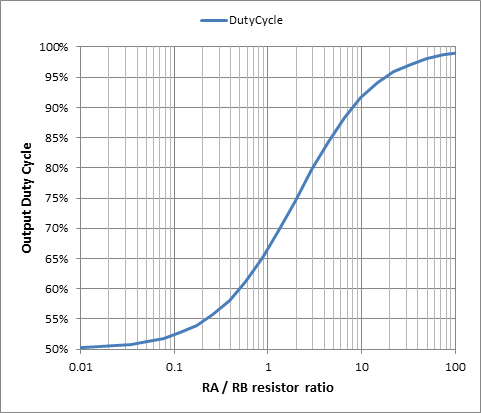Figure 2. Positive duty cycle versus RA/RB resistor ratio

The simple timing formula given by: 1.44 / [(RA + 2 * RB) * C], does not account for propagation delay. The error in the simple formula increases with higher a-stable frequency. The more complex formulas shown in Figure 3 can be used to calculate the expected low and high times more accurately. Propagation delays directly extend the end time of each output state. Propagation delay also creates a larger capacitor swing voltage, which increases the time needed for the next capacitor voltage to ramp. tPLH is the output low to high time delay and tPHL is the output high to low time delay. The graphic in figure 3 shows the effect of these output propagation delays.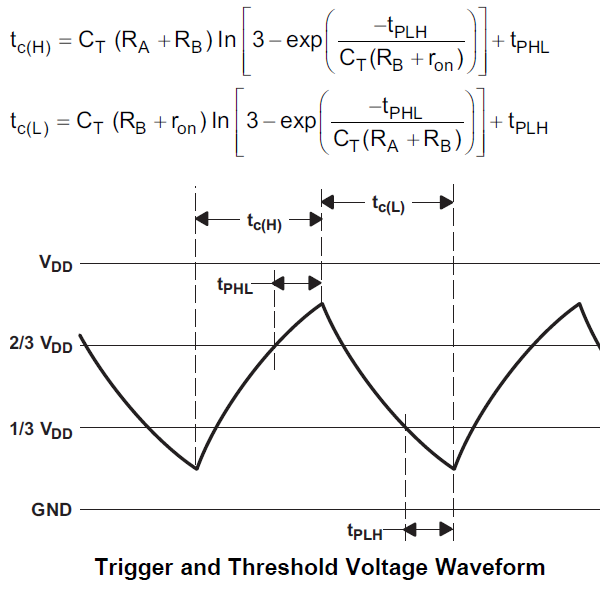Figure 3. A-stable frequency formulas including propagation delay where oscillation frequency will be 1 / [tc(H) + tc(L)]

Simple 50 percent duty cycle circuit

The output stage for the CMOS timers provide low and high output voltages, namely: VOL and VOH. These voltages approach the supply rails under light load. Therefore, a single resistor from output to timing capacitor can replace the two resistors that use the ‘discharge pin’. This provides nearly equal charge and discharge current flow. The VOH on the bipolar timers is significantly lower than the positive supply rail. So output duty cycle won’t be 50 percent.

The timing capacitor charges and discharges via the same resistor that is powered by the output VOH and VOL levels. This leaves the ‘discharge pin’ available as an alternate output. It is acceptable to leave the ‘discharge pin’ floating. The VOL drive is stronger than the VOH drive so the duty cycle is slightly higher than 50 percent. However, the variance from 50 percent duty cycle is insignificant when RC >> VOH driver resistance. The load on the output can also affect the frequency or duty cycle.

The simple formula to calculate oscillation frequency is 0.721 / (RC * C). The complete formula in Figure 3 can also be used by replacing (RB + rON) with (RC + ROL) and replacing (RA + RB) with (RC + ROH). ROL and ROH are the output resistances of the output VOL and VOH drivers respectively; the oscillation frequency will be 1 / [tc(H) + tc(L)].Figure 4. 50 percent duty cycle schematic for CMOS timers

Three resistor, a-stable circuit for 25 percent to 60 percent duty cycle

Adding a ‘discharge pin’ resistor (RD) will help reduce the output duty cycle because it increases the discharge resistance. RD lowers the discharging voltage magnitude due to the voltage divider of RA and RD. The discharge resistance is RB + (RA||RD) and the discharge voltage to supply voltage ratio is equal to 2/3 – RD/(RA+RD). The discharge ramp needed is 1/3 of the supply voltage. So the discharge time is C*(RB + RA||RD)*-ln(1-1/3/(2/3 – RD/(RA+RD)). This simplifies to C*(RB+RA*RD/(RA+RD))*ln((2*RA-RD)/(RA-2*RD)), which is still complicated. The charge time is the same as the standard setup, (RA + RB) * C* ln(2). This method works well for all types of timers.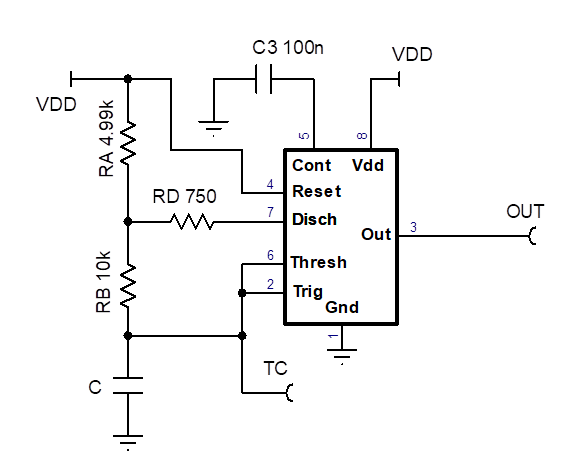Figure 5. Three resistors a-stable circuit

All three resistors (RA, RB and RD) control the output frequency and the duty cycle of this circuit, as shown in Figure 5. First choose (RA+RB) and C to get the desired output high time. Then choose one of the five RB/RA ratios [0, ½, 1, 2 and 10] plotted in Figure 6 and Figure 7. Finally, choose the RD/RA ratio needed to get the desired duty cycle.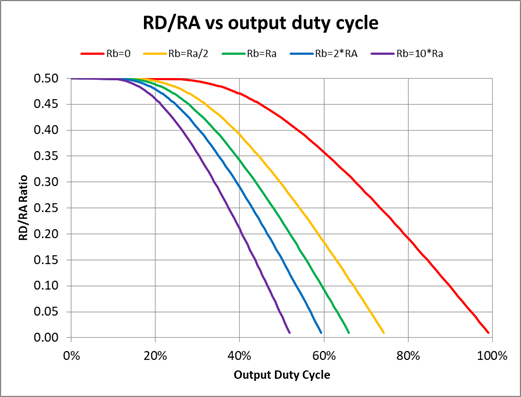Figure 6. Resistor ratios [0, ½, 1, 2 and 10] vs output duty cycle

Figure 7 shows the same data as Figure 6 except that the duty cycle range is limited from 25% to 60%. Duty cycles below 25% are omitted from the chart because the RD/RA ratio asymptotically approaches 0.5, as shown in figure 6. Duty cycles above 60% are omitted from this chart because the two resistor (RA and RB) circuit in Figure 1 is preferable for this output. Three common choices for scalars [RA, RB, RD] that produce a 50% duty cycle are [9, 9, 2], [1, 2, 0.15] and [2, 1, 0.59]. The last two sets fall nicely into standard 1% resistor choices.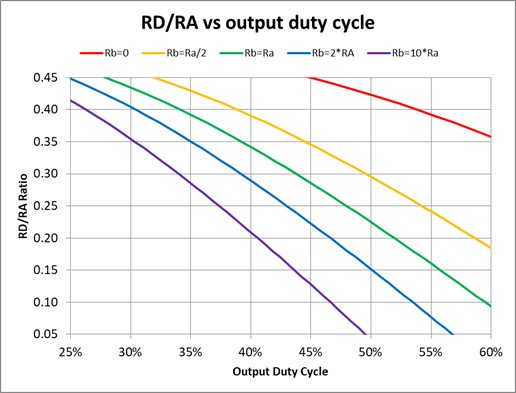Figure 7. Resistor ratios [0, ½, 1, 2 and 10] vs output duty cycle for recommended duty cycle range

Less than 50 percent duty cycle using a diode

In the standard circuit, the timer’s high time charges the capacitor through RA and RB (in series) and the low time discharges the capacitor through just RB. Therefore, the positive duty cycle can be between 50% and 100% exclusive. An additional steering diode can achieve lower duty cycle by bypassing RB. However, the voltage loss due to the diode is temperature sensitive; therefore the output duty cycle and frequency will have a greater temperature coefficient. Figure 8 shows an example of a circuit that includes a steering diode.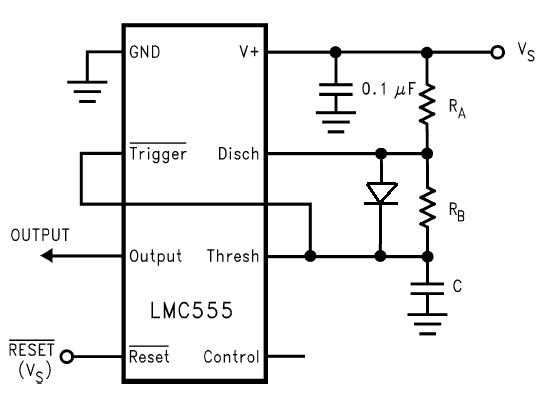Figure 8. Diode helps with output duty cycle below 50 percent

The output high time is set by RA and the diode voltage drop (VD), whereas the low time is set by RB. The capacitor, C, scales low and high time equally. Just like the standard a-stable circuit, the low time is RB * C * ln(2) and the high time is (RA + RB) * C* ln((2VS-3VD)/(VS-3VD)), where VS is supply voltage and VD is diode voltage. As supply voltage increases, the VD term becomes less significant. As supply voltage decreases, the VD term becomes more significant. For very low supply voltages, where VS < 3 * VD, the timer will never oscillate.

Texas Instruments provides a special timer tool to aid customers in their TLC555 timer design. The tool includes propagation delay (Tphl and Tplh) and discharge transistor on-resistance (Ron) to select components that will more closely match actual device performance. Default values for internal parameters reflect the TLC performance. For the other timers, set the “internal parameters” cells with values for the desired timer. This tool also supports adding a diode for low duty cycle output.Figure 9. TLC555 design calculator spreadsheet tool

The first a-stable pulse may be longer

Often overlooked is that the initial pulse high time will be longer than the successive high pulses. The first high pulse will be 59% longer, ln(3)/ln(2). Usually, the timing capacitor voltage starts at 0V at power up or after a reset release (similar to a mono-stable circuit) whereas all remaining cycles will start at 1/3 supply voltage. Figure 10 is basic a-stable timer circuit used for waveforms in figure 11.Figure 10. Power up schematic for simulation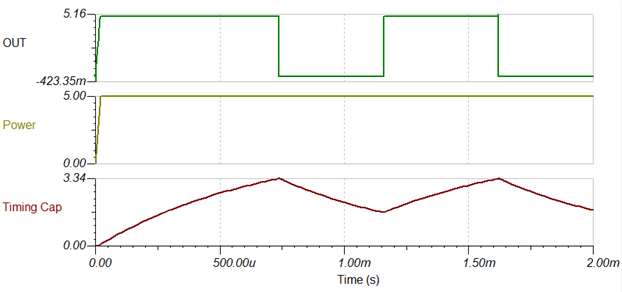Figure 11. Power up waveforms with standard RA, RB, and C setup.

The first high output will be the same width as the successive pulses if the control and timing capacitors are replaced with pairs of properly scaled capacitors (as seen in Figure12). The power up should be quick compared to the output high time, but not instantaneous (as seen in Figure 13). The two capacitor pairs create a capacitive voltage divider to bias the timing node to 1/3 VDD and control the node to 2/3 VDD at power up. The total timing capacitance is C = C2 + C3.

The original single capacitor helps isolate the timer comparators from noise that may be on the supply voltage pin. However, the capacitor pairs will couple supply noise to the timer comparators. 2/3 of VDD noise is present on the control pin and the threshold comparator; while 1/3 of VDD noise is present at the timing capacitor node and the trigger comparator. Note that the coupled noise can increase output pulse jitter.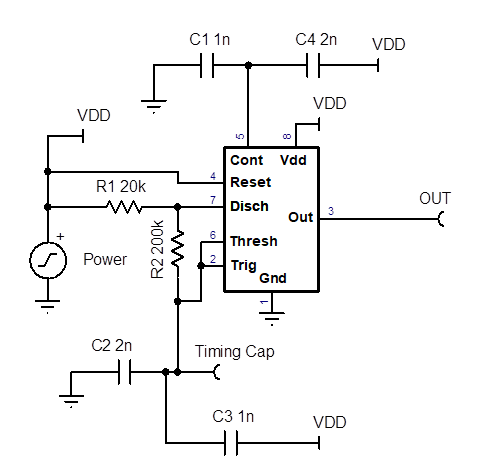Figure 12. Equal pulse width on power up schematicFigure 13. Power up simulation for C2 = C4 = 2nF and C1 = C3 = 1nF

Swapping capacitors C2 and C3 in Figure 14 will force the output to start at low level. The first pulse will have the same period and duty cycle as the successive pulses in Figure 15. The power up should be quick compared to output low time, but not instantaneous. The capacitor pairs create capacitive voltage dividers to bias the timing node and the control pin node to 2/3 VDD. The total timing capacitance is C = C2 + C3.

The two capacitor pairs will couple VDD noise to the timer comparators. 2/3 of VDD noise is present on the control pin, the threshold comparator and the timing capacitor node. 1/3 of the VDD noise is present on the trigger comparator. The coupled noise can increase output pulse jitter.Figure 14. Starts low with equal pulse width on power up schematicFigure 15. Power up simulation for C3 = C4 = 2nF and C1 = C2 = 1nF

A more complicated setup shown in Figure 16 eliminates the VDD noise coupling paths and supports reset pin toggling. At power up after a reset release, all output pulses will be the same.

R1 || RB and R3 generate a 1/3 VDD reference voltage that passes through T1, then to the timing capacitor, C, when the reset voltage is low. The reset input can be switched between 0V and VDD to control the output stream. The reset VIH, voltage input high, needs to be greater than 2/3 VDD to allow the timing capacitor to reach the threshold input level.

For a delayed power up start, add a capacitor to ground on the ‘Reset pin’. In order for T1 to have enough headroom to turn on, the VDD must be 3V at minimum. D2 and R4 will pull-up ‘reset pin’ voltage more quickly once the output goes high. D2 and R4 are recommended when a reset capacitor is used; these components are not needed for a clocked reset input signal.

As usual, RA, RB, and C set the frequency and duty cycle of the output stream. The timing capacitor pre-charge time is selectable. Choose a pre-charge time,‘t’, that is less than the minimum reset low time. Set R1 = t / (C * 2) where ‘C’ is the timing capacitance. Set R2 = R1 * 7 for a base current that ensures T1 to saturate. If VS > 8V, then use the base emitter protection diode, D1. An accurate value for R3 matters to get a 1/3 VDD reference while under the load currents of R1, R2, and RB. Calculate the factor ‘k’ = (VDD – 1.8V) / VDD, when D1 is not used and ‘k’ = (VDD – 3.6V) / VDD when the D1 diode is implemented. Then set R3 = 2 / (1/R1 + 1/RB + k/R2 ). T1 could be replaced with a PMOS, which makes the ‘k’ factor zero. With a PMOS transistor, R2 is optional and D1 diode cannot be used.Figure 16. Equal pulse after power up and reset release schematic.

Maximum Frequency

The CMOS data sheets have a typical value for the ‘maximum frequency’ specification.

In both cases, the test conditions use RA = 470Ω, RB = 200Ω, C = 200pf. These values would produce 8.3MHz on an infinitely fast timer. Therefore the actual frequency reflects the maximum frequency of timer. Only the TLC timer has a minimum value. The bipolar timers do not have a maximum frequency specification. Guidance is given by a chart in the ‘Typical Characteristics’ section of the data sheet. 100 kHz is the highest frequency on the chart.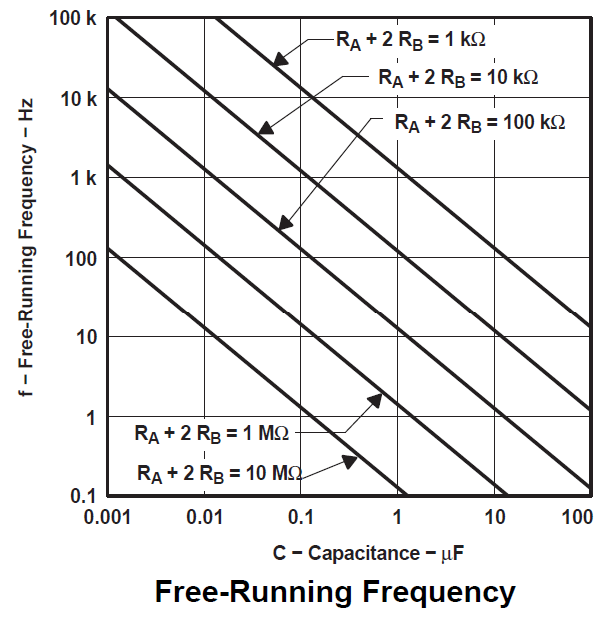Figure 17. Output frequency vs. timing capacitance, applies to bipolar and CMOS timers

Minimum Frequency

The minimum output frequency achievable is based on leakages in the timing capacitor, bias current and leakage in the timer’s ‘threshold pin’, ‘trigger pin’, ‘discharge pin’, and the PCB. The circuit board can be the biggest source of leakage if PCB condensation is possible. Leakage can also affect the accuracy of the frequency because leakage changes the effective capacitor charging and discharging currents.

The ‘threshold pin’ and ‘trigger pin’ in the bipolar timers will start to draw a bias current near both the threshold and trigger voltages as shown in the timing window lines of figure 18. Therefore, the bias current does not affect the charging current until the end of the capacitor voltage ramp. The charging resistor current needs to be greater than the threshold pin current. The CMOS timers do not have a bias current.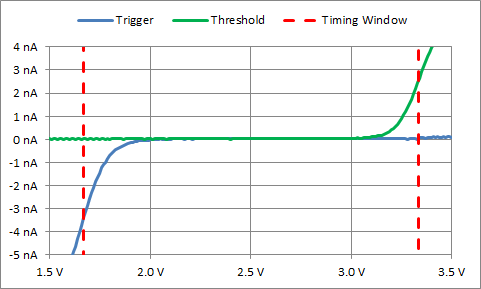Figure 18. Typical Bipolar ‘Threshold pin’ and ‘Trigger pin’ input bias current vs. pin voltage where Vs is 5V

Since a large capacitance is required to achieve a very low frequency, a nano-timer can be used as an alternative for low-frequency applications. They only require a single resistor to set the time delay up to 2 hours and use little power. Another alternative is to pass the timer output to a logic multi-stage ripple counter listed in table 1 to get a final very low frequency output with a small timer capacitance value. CD4060B has its own oscillator. So the 555 timer may not be needed.

 Part Number Maximum divider Number of outputs Includes oscillator CD4024B 128 7 No CD4040B 4,096 12 No CD4020B 16,384 12 No CD4060B 16,384 11 Yes

Table 1. Ripple counter options to extended minimum frequency or reduce timing capacitance.

Accuracy

In most cases, the tolerance of the timing capacitor will be the largest source of timing variation. Ceramic timing capacitors should be C0G/NP0 type for the best temperature coefficient, voltage modulation, and aging characteristics. The timing resistors’ tolerance and temperature coefficient is also important. The higher the application frequency, the more propagation delays will affect output frequency.

The initial a-stable timing accuracies are typically 1 percent for TLC, 1.5 percent for SE, 2.25 percent for LM, NA, NE, SA, and unspecified for LMC. The maximum frequency error is 3 percent for TLC and 17 percent for LMC, which is very pessimistic. All accuracies are based off 25°C values and temperature coefficients in the data sheets are only typical values. For full temperature range final accuracy better than 5 percent, the cost of improving the timing capacitor and resistor may be higher than the cost of a crystal oscillator and a logic multi-stage ripple counter.

LMC555, calculations [4.0/4.8-1 = -17% ; 5.6/4.8-1 = +17%] (datasheet revision M)NA/NE/SA/SE (data sheet revision I)Control voltage

The ‘control’ pin voltage is internally generated and is normally 2/3 of the VS. The control voltage can be forced to a different voltage. Both the frequency and duty cycle will change with control voltage. Adding a capacitor to the control pin helps reduce jitter when the supply voltage is noisy. On power up the control capacitor will lag the supply voltage based on the impedance of the control pin and the control capacitor. The data sheets do not specify control pin impedance; refer to table 2 for sample data ‘control pin’ impedance, temperature coefficients, and voltage coefficients at 25˚C and VS = 5V

 Timer Impedance Temperature Coefficient Supply Coefficient Units kΩ %/˚C %/V LMC 60 0.75 0.39 TLC 52 0.48 -2.60 LM 3.3 0.15 0.06 NA, NE, SA 3.3 0.18 0.08 SE 1.6 0.16 0.05

Table 2. Sample data ‘control pin’ impedance at 25˚C and VS = 5V

Reset input

When the ‘reset pin’ voltage goes low, the output will go low as well. The ‘discharge pin’ will also go low and the timing capacitor will discharge through the resistor RB. The output will remain low until ‘reset pin’ is returned to high. When reset goes high, the output will go high if the timing capacitor voltage is below 1/3 VS. Otherwise, the output will remain low until the timing capacitor voltage drops to 1/3 VS.